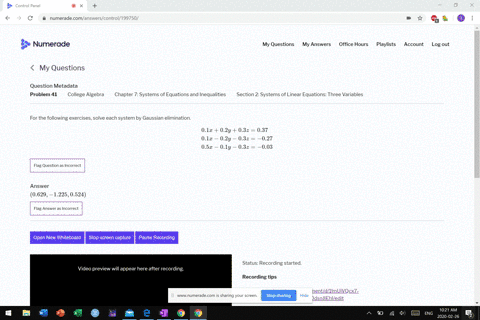Sign up for our free STEM online summer camps starting June 1st!View Summer Courses### For the following exercises, solve each system by…

02:32
MB

Need more help? Fill out this quick form to get professional live tutoring.

Get live tutoring
Problem 40

For the following exercises, solve each system by Gaussian elimination.
$$\begin{array}{l}{0.5 x-0.5 y+0.5 z=10} \\ {0.2 x-0.2 y+0.2 z=4} \\ {0.1 x-0.1 y+0.1 z=2}\end{array}$$

the system has no solution.

## Discussion

You must be signed in to discuss.

## Video Transcript

All right. Question. 40 three wages, three unknowns. So by galaxy elimination, and in this case, we have two decimals. Um, but notice that each problem has a lot of step. Mom s O, for instance, 25.5 point five. And so basically, I'm Regis. Divide this by 0.5. And so I get X minus. Why? Plus Z equals 20. This one right here. I divide this by 0.2 and I get X minus Y plus Z equals 20. And then finally this one divided by 10.1. You guessed it get X minus Y buzzy. It's 20. These are all the same plane all of you claim. So there's no you unique solution. Matter of fact, there is an infinite a number of solutions. You said that works in one. We're working them out